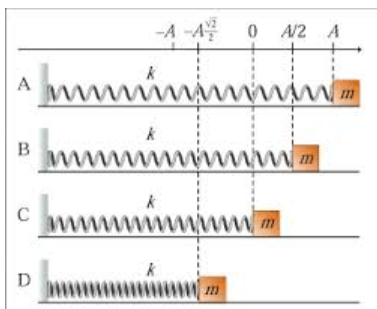# Problem: Figure 1 shows a harmonic oscillator at four different moments, labeled A, B, C, and D. Assume that the force constant k, the mass of the block, m, and the amplitude of vibrations, A, are given. We will also assume that there are no resistive forces so the total energy of the oscillator remains constant.Find the kinetic energy of the block at the moment labeled B.

###### FREE Expert Solution

Angular frequency:

$\overline{){\mathbf{\omega }}{\mathbf{=}}\sqrt{\frac{\mathbf{k}}{\mathbf{m}}}}$

Spring potential energy:

$\overline{){\mathbf{U}}{\mathbf{=}}\frac{\mathbf{1}}{\mathbf{2}}{\mathbf{k}}{{\mathbf{x}}}^{{\mathbf{2}}}}$

83% (415 ratings)###### Problem Details

Figure 1 shows a harmonic oscillator at four different moments, labeled A, B, C, and D.Assume that the force constant k, the mass of the block, m, and the amplitude of vibrations, A, are given. We will also assume that there are no resistive forces so the total energy of the oscillator remains constant.

Find the kinetic energy of the block at the moment labeled B.

Frequently Asked Questions

What scientific concept do you need to know in order to solve this problem?

Our tutors have indicated that to solve this problem you will need to apply the Intro to Simple Harmonic Motion (Horizontal Springs) concept. You can view video lessons to learn Intro to Simple Harmonic Motion (Horizontal Springs). Or if you need more Intro to Simple Harmonic Motion (Horizontal Springs) practice, you can also practice Intro to Simple Harmonic Motion (Horizontal Springs) practice problems.